# Multiplicative group of a prime field is generated by all primes less than square root plus one

## Statement

Supposeis an odd prime. The multiplicative group modulois generated by the congruence classes of all primes less than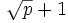.

## Related facts and conjectures

• Smallest quadratic nonresidue is less than square root plus one
• Extended Riemann hypothesis: A stronger form of the Riemann hypothesis that is equivalent to the statement that the smallest quadratic nonresidue modulo, for any odd prime, is less than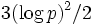, wherehere is the natural logarithm.
• Artin's conjecture on primitive roots: This is a closely related conjecture that states that every integer that is notor a perfect square occurs as a primitive root for infinitely many primes.

## Proof

Given: An odd prime,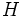is the subgroup of the multiplicative group modulogenerated by all primes less than.

To prove:is the whole multiplicative group modulo.

Proof: Note first that any positive integer less thanis a product of primes less than. Hence, its congruence class is in.

Letbe the smallest positive integer whose congruence class is not in. Then,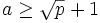.

Letbe the smallest positive integer such that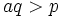, and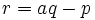. Sinceis prime,, so. Further, we have: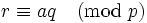.

Sinceis not in, either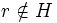or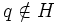. However, since,, while. Thus, we have found a positive integer smaller thanthat is also not in, contradicting minimality of.